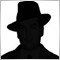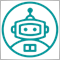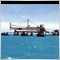• 概述
• 评论 (4)
• 评论 (15)
• 新特性

# Synthetic

This is an arbitrage indicator.

The indicator displays the chart of an arbitrary combination of trading instruments, described by the entered arithmetic formula.

Using the indicator.

1. Add an indicator to any chart.
2. Enter the formula as a string in the indicator's parameters. All symbols used in the formula must exactly match the names in the table "Market Overview" of the terminal. Example of the formula:
3. `(SIBN + SNGS) / 'RTS-3.19' + 2.5 * GAZP / LKOH`
4. Start the indicator

The formula must meet the following requirements:

• The names of the symbols must exactly match the names in the table "Market Overview" of the terminal.
• In the formula are allowed:
• arithmetic operations: '+', '-', '*', '/';
• numerical constants;
• brackets.
• The names of instruments containing arithmetic operations symbols (such as 'RTS-3.19') must be concluded in single quotes ('RTS-3.19').
• The spaces in the formula are ignored.[删除] 2019.01.14 08:0112010
2018.01.14 17:585715
2017.11.09 14:336208
2016.03.25 00:23

Fix Errors

In the formula are allowed:
- arithmetic operations: '+', '-', '*', '/';
- numerical constants;
- brackets.
The names of instruments containing arithmetic operations symbols (such as 'RTS-3.19') must be concluded in single quotes ('RTS-3.19').

Fixed the shift of the indicator chart relative to the main chart on the history data

It is now possible to add ratios before instruments. Ratios can only be integers, only used as multipliers.